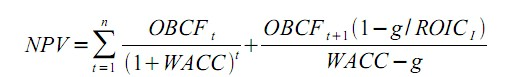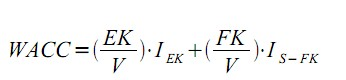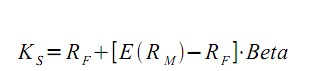# How to use wacc and npv

## Net present value

### Definition: what is the net present value?

The Net Present Value (NPV), also known as the net present value, is a method for evaluating long-term investment projects.

### Basics of Net Present Value

Net present value is the discounting of future investment income from an investment to the current point in time. The result is a monetary key figure in the correspondingly calculated currency. As soon as the net present value is positive, the investment project generates more value than a risk-adjusted minimum return on the capital market, which consequently leads to an increase in shareholder value.

One problem with the evaluation of investment projects is their indefinite lifespan. A solution that is often used for this is to divide the investment value into two different periods. The first period reflects the present value of the cash flows during the detailed forecast. The second period, on the other hand, shows the present value of the cash flows according to the detailed forecast. The last-mentioned period is also referred to as the continuing value.

In the following formula for determining the net present value, the first fraction represents the detailed forecast and the second fraction represents the continuation value for calculating the net present value:Figure 1: Formula Net Present Value

OBCF = gross operating cash flow

WACC = Weighted Cost of Capital

n = The last year of the detailed forecast

t = time

OBCF (t + 1) = average rate for the first year after the period of the detailed forecast

g = growth rate

ROIC (t) = Additional return on the newly invested capital

### The detailed forecast

In the detailed forecast, the present value of the gross operating cash flows corresponds to the discounted value of the gross operating cash flows expected for the future. The gross operating cash flow is determined according to the following scheme:

Sales
- manufacturing costs
= Gross profit

- Research and development costs
= EBIT

= Gross operating cash flow (EBITDA)

The EBITDA must now be discounted with the weighted average cost of capital (WACC) in order to match the definition of the detailed forecast. The level of the discount rate should include the opportunity costs of the lenders and equity providers involved in the investment project. For this purpose, the return claims of the equity capital providers as well as the interest on the borrowed capital must be defined. As a result, the cost of capital - compared to accounting performance indicators such as profit - includes the cost of rewarding all the investors involved.

The weighted cost of capital can be calculated using the following formula, also known as the total cost of capital:Figure 2: Weighted cost of capital formula

EK = equity value

V = company value (total capital value)

I (EK) = cost of equity

FK = debt capital value

I (S-FK) = average rate for the first year after the period of the detailed forecast

In practice, the determination of the cost of equity turns out to be difficult because the interest claims are not contractually stipulated. There are different approaches to determining the cost of equity, such as the Capital Asset Pricing Model (CAPM), the Arbitrage Pricing Theory (APT), the Option Pricing Model (OPM) or the Coherent Market Hypothesis (CMH).

Tom Copeland, author of the book "Valuation", recommends the capital asset pricing model and the arbitrage pricing model for determining the opportunity costs of equity. The first is briefly presented below:

The CAPM was originally developed for investors in the stock market. However, it can be transferred to companies to determine the cost of equity without further problems. Equity providers invest capital and expect a certain rate of return for it. If the investment were not associated with any risk, the return would be the risk-free interest rate, e.g. the amount of a federal bond. However, since an investment is usually associated with risk, equity investors charge an additional risk premium that corresponds to the level of risk. This additional premium for the risk is determined by multiplying the difference between the general market return and the risk-free interest rate by the beta factor.

The market return is usually derived from a representative market index. The beta factor, as a measure of the systematic risk, shows how much the return on the investment fluctuates around the market return. Publications in financial magazines or portals can be used to determine the beta factor for stock corporations listed on the stock exchange.

However, if the beta factors from different sources differ by more than 0.2 percentage points or the beta factor differs by more than 0.3 percentage points from the industry average, it is advisable to use the values ​​of the industry average for calculating the cost of equity use. For companies that are not listed on the stock exchange, you should always orientate yourself according to the industry average. This relationship is expressed by the following formula:Figure 3: Formula cost of equity

K (s) = cost of equity

R (F) = Risk Free Return

E (R [M]) = expected value of the return on the market portfolio

E (R [M]) - R (F) = risk premium

Beta = systematic risk

As mentioned before, in order to determine the WACC, in addition to the opportunity costs of the equity capital provider, the opportunity costs of the debt capital provider must also be taken into account. The expectations of the lenders with regard to the interest rate can be derived from the contractually defined interest rate agreements and are therefore very easy to determine within the company. However, if the occasion requires the determination of the external capital costs of an external company, these can be derived approximately from the income statement, provided that the interest expense is stated there.

### The going concern value

In the second period to determine the net present value, the present value of the cash flows is determined according to the detailed forecast. There are different formulas for determining the going concern value, some of which are based on restrictive assumptions or partly on unrealistic assumptions. The above formula for the going concern is what is known as the “value driver formula” recommended by Copeland as it relates to economically sensible assumptions that are included in the company's analysis. The estimation of the various variables of the going concern value must be made with careful care, as this often makes up a large proportion of the total value of the investment project.

For example, care should be taken to ensure that the estimated free cash flows match the expected growth rate. It is normally the case that the expected growth in the period of the going concern value is lower than the growth in the period of the detailed forecast. As a result, a smaller portion of the operating profit after tax has to be invested in order to ensure growth. If this relationship is not taken into account, the consequence is that the going concern value will be underestimated.

When estimating the expected return on the new investment, it should also be ensured that these are in accordance with the expected competitive conditions, as empirical studies have shown that a company cannot normally achieve above-average returns under competitive conditions. This means that the return on invested capital (ROI) should be equal to the weighted average cost of capital. The net present value is also adequately reflected without a going concern value if a conservative estimate of the investment value is intended or if no further values ​​are generated by the investment after the detailed forecast has expired.